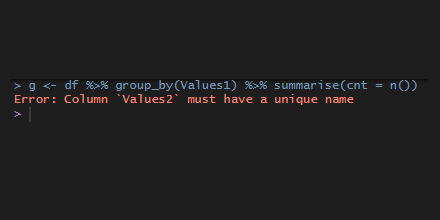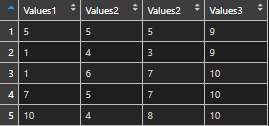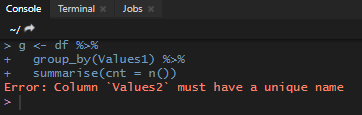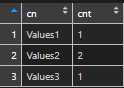# dplyr error: column must have a unique nameIn rare cases, the data source may contain multiple columns with the same name, and some of the next actions with dplyr functions might result in the error “column must have unique name”.

Here is a data frame with columns that have the same name.

```df <- data.frame(
Values1 = sample(1:10, 5, replace=T),
Values2 = sample(1:10, 5, replace=T),
Values2 = sample(1:10, 5, replace=T),
Values3 = sample(1:10, 5, replace=T),
check.names = FALSE
)```If I want to group and summarize something, I get this error.

```g <- df %>%
group_by(Values1) %>%
summarise(cnt = n())```To move one, you have to rename some of the data frame columns.

In large data frames, a summary of data frame column names might be handy.

```name_count <- data.frame(cn = names(df)) %>%
group_by(cn) %>%
summarize(cnt = n())```